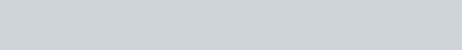# Python switch case

Filed Under: Python

Dear learners, hope that you are learning well. In our previous tutorial you learned about Python Pickle. In this tutorial we will look into python switch case statement.

## Python switch case

Though many other languages offer switch statements, python does not have any switch statement. According to PEP-3103, it assure about the fact. However if you like switch case statement in other programming languages, you can create code snippet that works like a switch case statement in python.

### Using Dictionary to Return value

You can use dictionary to work like a switch statement. Here the keys of the dictionary will work as `case`.

Then according to the keys, the value of the dictionary will work as the result. We will give some example through out this tutorial. See the following code to understand this simple example of switch case statement alternative. See our Python Dictionary tutorial, if you don’t know about Python dictionary.

``````
b ={
'a' : 122,
'b' : 123,
'c' : 124,
'd' : 125
}

# take user input
inp = input('input a character : ')

# -1 is the default value if there is no keys that matches the input
print('The result for inp is : ', b.get(inp, -1))

``````

So for my case I entered ‘b’ as input. The output was following### Python switch case statement alternative with dynamic functions

In this section we will see how to perform dynamic action based on dictionary keys. So, imagine a scenario where we need to calculate different thing based on the input. How to do that using dictionary? Well, in this case we will use Python Lambda function to do that. See the following example as an alternative of python switch case statement.

``````
def switch_func(value, x):
return {
'a': lambda x: x+122,
'b': lambda x: x*2,
'c': lambda x: x-123,
'd': lambda x: x/2
}.get(value)(x)

# take user input
inp = input('input a character : ')

print('The result for inp is : ', switch_func(inp, 2))
``````

I used ‘d’ as input. So the output of the following code was like this

``````
input a character : d
The result for inp is :  1.0
``````

So, that’s all about Python switch case statement alternatives. It’s not recommended to use this kind of code snippet. Python if else technique is more elegant to use than this snippet. But it is never bad to learn about new techniques.

1.Abhishek Madhu says:

Python 3.10 introduces match-case, which is similar to switch case.
Here is a short video describing how that works.

https://youtu.be/Z16yW2VmpL4

The official Python Documentation PEP link can be found in the video description.

Many thanks.